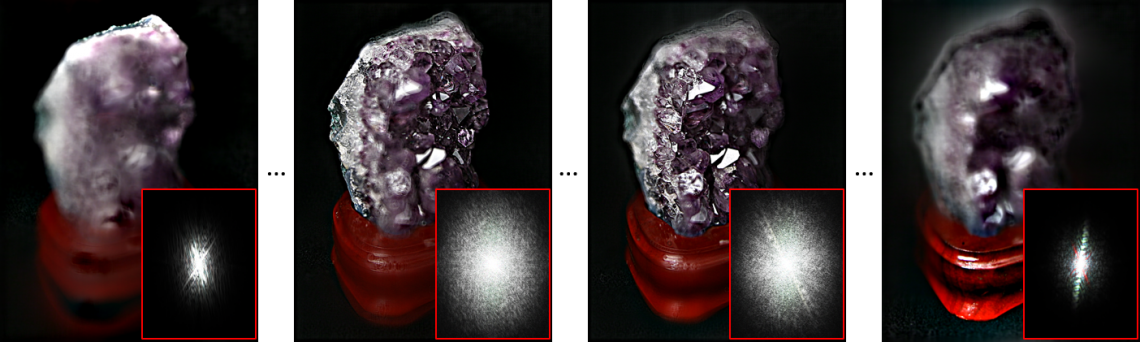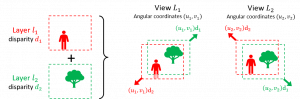# A Fourier Disparity Layer representation for Light Fields

31st May 2019## Principle

The Fourier Disparity Layer (FDL) model, is a representation of Light Fields allowing efficient processing by fully exploiting the parallelisation capabilities of modern GPUs.

In the FDL representation, the scene is decomposed into a set of additive layers and each layer of index k is associated to a disparity value dk representing its depth. Any view of the light field at angular coordinate (u,v) is directly reconstructed as the sum of the layers shifted with a translation vector (u.dk, v.dk).The construction of layers from light field views is performed efficiently in the Fourier Domain thanks to a linear least squares optimisation problem formulated independently for each frequency component (efficiently solved with parallel processing on a GPU). This is made possible by the fact that in the Fourier domain, the shifting operation simply consists in multiplying each frequency component by a complex weight, hence resulting in a linear relationship between views and layers.

## Publications

• The paper , presents the theoretical foundations of the model and various applications including calibration of angular coordintates and disparity values, view interpolation, denoising, real-time rendering (see demo video below).
• Extensions of the FDL construction for light field super-resolution, demosaicing and completion applications are presented in . By performing completion jointly with super-resolution and demosaicing, the method also allows the extraction of high quality views from RAW lenslet data of plenoptic cameras (see examples of results below).
• The compression of light fields is addressed in  with a view prediction scheme based on the FDL view interpolation.
• Another light field compression and streaming method that directly encodes the FDL model is presented in . It constructs a binary tree structure from the layers to encode the FDL in a hierarchical manner, thus providing a scalable representation for light field streaming.

## Code

The matlab code is available on github: here!

## Results

Demonstration of the applications in